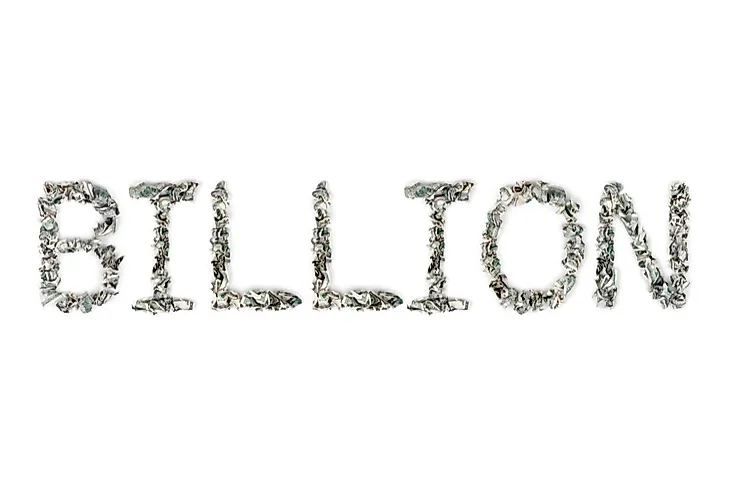# How Many Zeros Are in a Billion?There are two methods that can be used to determine the number of zeros in a billion.

Have you ever considered what number of zeros are in a billion? The number of zeros of in a billion is subject to what part of the world you come from and your age to a certain extent, because it influences the system that was used at the time.

There are two main methods of determining the number of zeros in a billion. These are known as the short system and the long system.

## Long Scale and Short Scale

Both Britain and the United States use the short system of naming the value of a billion. This is also common in most other English-speaking countries across the world. The short scale system was adopted in Britain in 1974 and continues to be used today. In the short scale system, one billion = one thousand million, which is the same as 1 and 9 zeros. The short scale method of determining the number of zeros in a billion is mainly used in the financial and scientific fields. This helps in simplifying the figures.

On the long scale method, a billion= one million million, which is equivalent to 1 and 12 zeros. The long scale system is used in various countries in Europe such as France, Germany, Norway, and multiple Latin America countries. Canada (a bilingual nation) uses both scales, each for multiple dialects.

The long scale is a large-number naming system that uses powers of one million, whereas the short scale used powers of a thousand. For numbers below a thousand million, both scales are similar but change once the number values exceed a thousand million. The short scale is used in English speaking countries and Arabic countries whereas the long scale is dominant in Europe, Spanish speaking countries, and Portuguese-speaking countries except for Brazil. In this regard, it is essential to differentiate between the two scales when comparing large numbers in different countries which might still be using the long scale system.

## The Short Scale

Ten: 10 (1 zero)

Hundred: 100 (2 zeros)

Thousand: 1000 (3 zeros)

Ten thousand 10,000 (4 zeros)

Hundred thousand 100,000 (5 zeros)

Million 1,000,000 (6 zeros)

Billion 1,000,000,000 (9 zeros)

Trillion 1,000,000,000,000 (12 zeros)

A Billion (power of base ten)

109 = 1,000,000,000

10 x 10 x 10 x 10 x 10 x 10 x 10 x 10 x 10 = 1,000,000,000

A Billion (power of base a thousand)

1 Billion= 10003 (Short scale)

1000 x 1000 x 1000

## The Long Scale

One (1,000,000)0

Million (1,000,000)1

Billion (1,000,000)2 = 1,000,000 x 1,000,000 = 1,000,000,000,000

Trillion (1,000,000)3